# Gmat Data Sufficiency – Seeing the Big Picture

Gmat Data sufficiency is annoying to many students because of its unfamiliar format. In exams, we are used to being directly asked what to do. For example, solve for x, or find the Area. It is a direct order. However, there is some uncertainty with Data Sufficiency that can be annoying to some.

Compare the following two situations:

Being asked to “find x” vs “is x positive?”

The former is a simple and direct order: There is some unknown x, find it.

The latter seems to involve many scenarios. This uncertainty is uncomfortable for many. Compare this to a problem in real life. Your boss asks you to do something, for example “Here are some numbers. Make them into a graph” vs. “what numbers should we use? do some research”. There is more imaginative work required in the second question and more autonomy to decide what to do.

Problem solving questions are “how” questions, whereas data sufficiency are “why” questions. “Why” questions are always more difficult. If you struggle with Data sufficiency, it is perhaps because you prefer order, structure and clear directions. This is not bad, as it will serve you well in the exam because structure prevents silly mistakes and saves time. But you should complement this preference for structure with more curiosity. Understand the situation that the problem presents, define its boundaries, and divide it into mini problem solving questions that you can solve easily.

## The Gmat data sufficiency solving strategy

• Know the theory well, inside out. If your understanding of the theory is mediocre or incomplete, you will miss the subtleties, exceptions, and tricks.
• Understand the structure of Data Sufficiency. When you begin to analyze statements (1) & (2), you should assume that they are true.
• Read the question carefully and understand what they ask. Visualize the problem.
• Highlight the relevant theory in your mind. For example, if the question is about evens or odds, immediately remember the rules, especially the special cases; for example, that zero is even, but neither positive nor negative.
• Read statement (1) alone, assume it is true, and determine if the question can be solved. Scenario planning. Pan out the different possible outcomes.
• Read statement (2) alone, assume it is true, and determine if the question can be solved. Scenario planning. Pan out the different possible outcomes.
• Proceed to determine whether they are sufficient together or not.
• Remember: You do not need to solve the question, you only need to know whether or not it can be solved.

Take a look at the following question: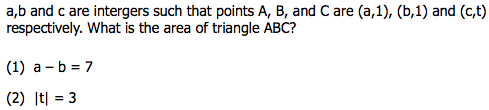1. understand the question and visualize it. There are 3 points on a plane that form a triangle. Two of the points are on the same horizontal line (y-coordinate of a&b = 1) and there is a certain point C elsewhere in the plane. What is the area of the triangle they form? Draw what you already know.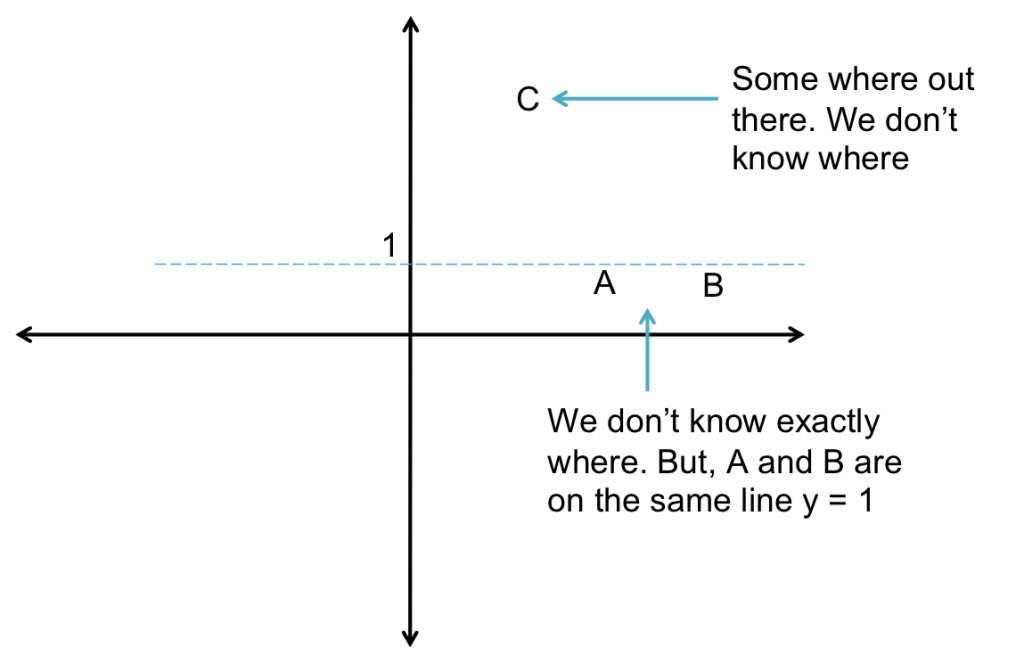2. Highlight the relevant theory in your mind: Area = (1/2) x Height x Base
3. Read statement (1), and perform the scenario planning. Sufficient by itself?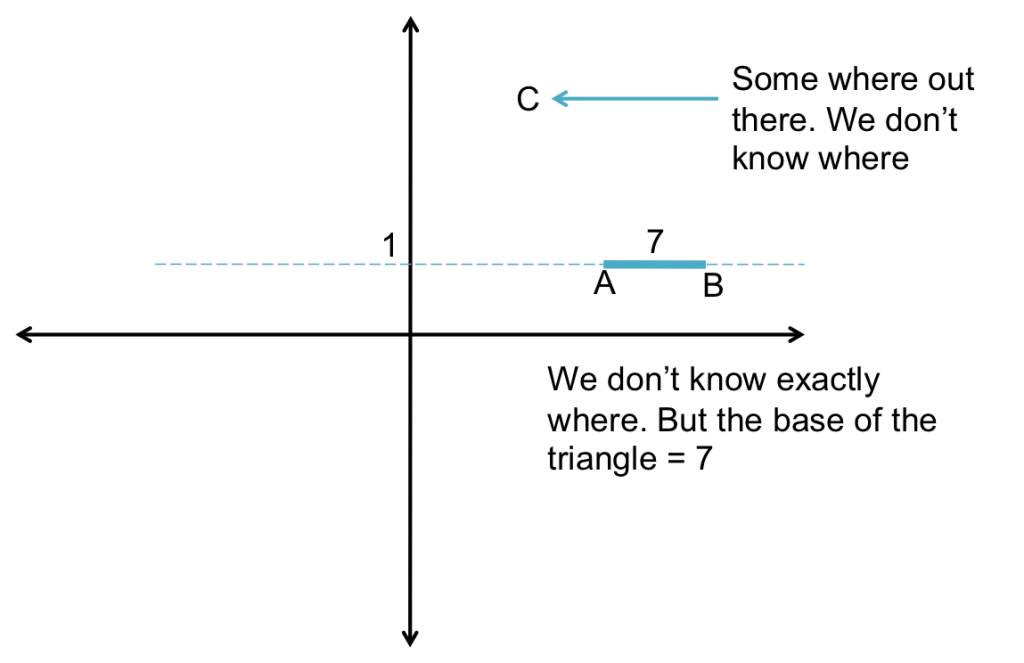4. Read statement (2), and perform the scenario planning. Sufficient by itself?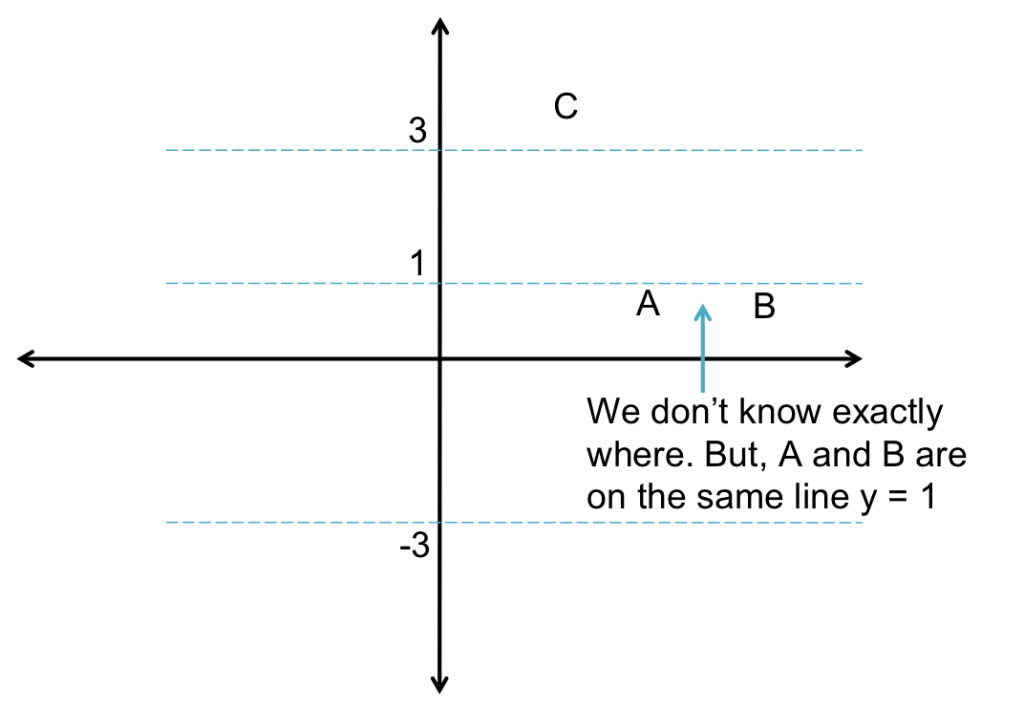5. Determine if together they are sufficient or not.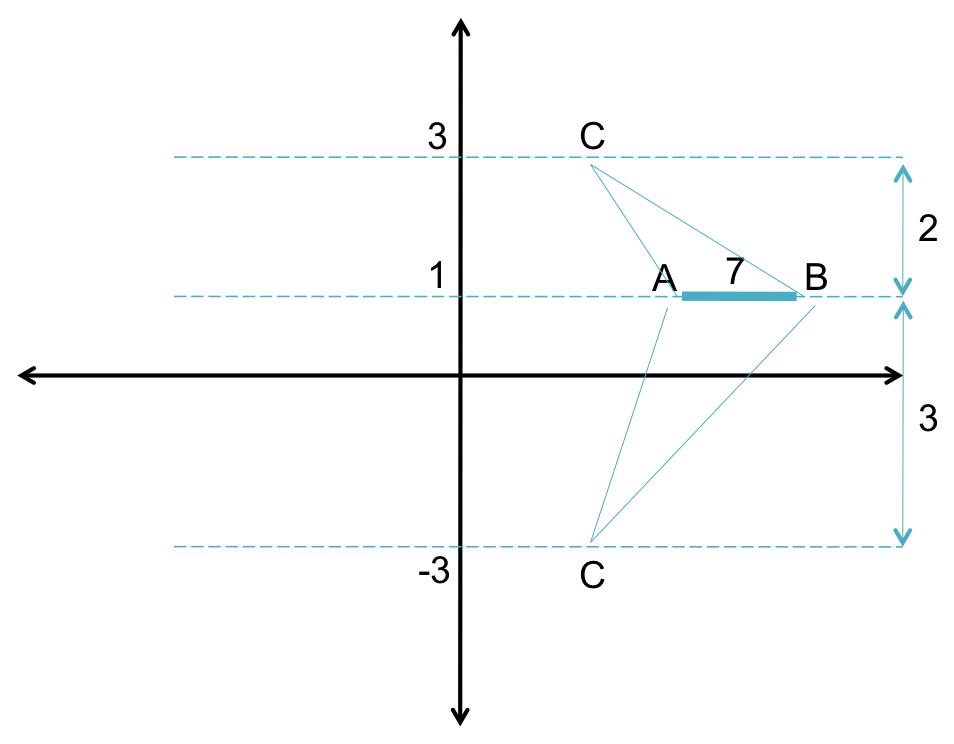Notice what we have done. After reading and understanding the question, we visualized it, and proceeded to determine the different scenarios possible based on statements (1) & (2) alone, and then together. Statements (1) & (2) alone are not sufficient. Putting them together, results in the final figure above. Now we narrowed down the situation to two scenarios; one where the height of the triangle is 2 and another where the height of the triangle is 3. We need one answer, not two possible answers.

Hence the answer to this question is E.

Note:

1. Given that the base = 7, we don’t care where exactly A and B are on the horizontal line y = 1.
2. Had the points A and B been on the line y = 0, the answer would have been (C), because the height would be = 3. In that case, it wouldn’t matter where the point C is on the lines y = -3 or 3, because the height of triangle ABC will always be 3. Remember, that the area of a triangle = (1/2) x Height x Base.

It may seem like a lot of work, but during the test, you won’t have to draw all four figures. You draw just enough to visually represent the facts given and determine the different scenarios possible.

When you internalize this process, you will realize that Gmat Data Sufficiency questions are more fun, like a puzzle in fact. The best part, you don’t need to solve the problem, you just need to know whether or not you can.

This site uses Akismet to reduce spam. Learn how your comment data is processed.